# Influence of thermal-mechanical coupling effect on vibration of double-drive feed system

Influence of thermal-mechanical coupling effect on vibration of double-drive feed system

Jun HuanJuntang Yuan Zhenhua Wang

School of Mechanical Engineering, Nanjing University of Science and Technology, Nanjing, 210094, P.R. China

Corresponding Author Email:
huangjun_happy2006@163.com
Page:
177-182
|
DOI:
https://doi.org/ 10.18280/ijht.350123
|
Accepted:
|
Published:
31 March 2017
| Citation

OPEN ACCESS

Abstract:

A double-drive feed system has the characteristics of complex loads and multi-thermal sources, so the dynamic performance of the feed system is a thermal-mechanical coupling problem. In this paper, the mechanism of the thermal-mechanical coupling effect on the vibration of the machine tool feed system is discussed. The effect of the thermal-mechanical coupling effect on the dynamic performance of the feed system was analyzed by experimental data, as well as the influence of the coupling effect on the structural deformation. The above results showed that a rising temperature made the natural frequency of the system decrease and the vibration amplitude of the feed system increase with a maximum rate of increase up to 14.06％. Through experiment and simulation, the dynamic simulation and analysis method of the thermal- mechanical coupling of a machine tool feed system are shown to be rational and feasible.

Keywords:

Thermal Field, Thermal-Mechanical Coupling, Double-Drive Feed System, Vibration

1. Introduction

In recent years, research on solving the phenomenon of machine vibration has mainly depended on improving the stiffness of machine tools. In general, machine tool stiffness includes static stiffness, dynamic stiffness and thermal stiffness. Dynamic stiffness is an important factor affecting the structure of the machine tool due to the change of cutting force . Thermal stiffness is the ability to resist heat distortion. In a precision, numerical control machine tool, the thermal error accounts for 60% ~ 70% of the total errors . In addition, thermal-mechanical coupling stiffness has a great influence on the dynamic performance . Therefore, research on the vibration of the machine tool is the problem of actual dynamic stiffness and thermal-mechanical coupling stiffness  . Figure.1 shows the structure of machine tool’s feed system.

## 1.png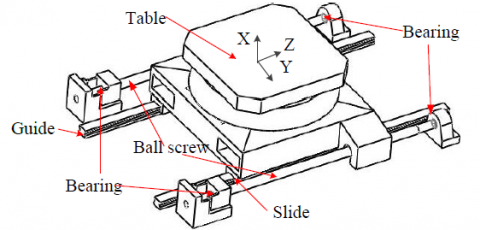Figure 1. Double-drive feed system

Double-drive technology puts the movement of the components between the two ball screws and forms an ideal and virtual center of gravity. It is an excellent way to restrain the vibration and bending of the ball screws, even if the movement components are in high-speed conditions. The center of gravity will not change; thus, they achieve a stable drive . The center of gravity driving is a technology based on the development of mechanical motion dynamics theory. Y Tian  thought that the driving force in the machine process is not very accurate at the center of the movement. Therefore, at a high cutting speed, especially in larger feed rate conditions, there is a trend to reverse the movement.

Up to now, research on the double-drive feed system has mainly concentrated on dynamic performance and thermal performance. YangJ  analyzed the temperature field and thermal deformation of the double-drive feed system of the machine tool under different working conditions, and obtained the ideal prediction results. Heiseu  measured the temperature field by an infrared thermal imager, and the relationship between the thermal error of the feed system and the temperature of the key points has been established. However, the vibration and thermal analysis of the feed system are mostly confined to the structure field, which ignores the effect of thermal-mechanical coupling on the vibration of the feed system during the machining process, and it is difficult to reflect the vibration performance of the feed system. In this paper, the influence of thermal-mechanical coupling on the dynamic performance is discussed. Additionally, the comparison between simulation and experiment is analyzed.

2. Mathematical Model

2.1 Heat source analysis of double-drive feed system

The main heat sources of the double-drive feed system are as follows: (1) motor heating power, (2) rolling guide rail and the slider friction heat, (3) ball screw and nut friction heat, (4) bearing friction heat. As is shown in Fig.2, the heat transfer model is complex due to the friction and combination of the components in a double drive feed system. In this model, the sources of heat are composed of four parts: two servo-motors, four guide rail-sliders, two screw-nuts, and four ball bearings, followed by Q1, Q2, Q3 and Q4. It is necessary to study the capacity of heat sources and the temperature, which is a vital basis for investigating the thermal force coupling mechanism of the double-drive feed system.

## 2.pngFigure 2. Thermal source of double-drive feed system

2.2 Temperature field analysis theory

Generally, the finite element method and finite volume method were used to simulate the temperature field of a CNC machine tool feed system . In this paper, the finite element method is used. The temperature field of double-drive feed system analysis of the finite element and the equation are:

$[{{C}_{t}}]\{\overset{.}{\mathop{T}}\,\}+[{{K}_{t}}]\{T\}=\{Q\}$          (1)

For the temperature field of the double-drive feed system, the elements of [Kt], [Ct] and {Q} are obtained by the corresponding matrix elements.

${{K}_{ij}}=\sum\limits_{e}{K_{ij}^{e}}+\sum\limits_{e}{H_{ij}^{e}}$           (2)

where

$K_{ij}^{e}=\int_{{{\Omega }^{e}}}{({{\lambda }_{x}}}\frac{\partial {{N}_{i}}}{\partial x}\frac{\partial {{N}_{j}}}{\partial x}+{{\partial }_{y}}\frac{\partial {{N}_{i}}}{\partial y}\frac{\partial {{N}_{j}}}{\partial y})d\Omega$,

$H_{ij}^{e}=\int\limits_{S_{s}^{e}}{\alpha {{N}_{i}}{{N}_{j}}dS}$

${{C}_{ij}}=\sum\limits_{e}{C_{ij}^{e}}$          (3)

where,

$Pi=\sum\limits_{e}{p_{qi}^{e}}+\sum\limits_{e}{p_{Hi}^{e}}+\sum\limits_{e}{p_{Qi}^{e}}$          (4)

where

$p_{qi}^{e}=\int\limits_{s_{2}^{e}}{{{N}_{i}}}qdS$, $p_{Hi}^{e}=\int\limits_{s_{3}^{e}}{{{N}_{\alpha }}}{{T}_{\alpha }}dS$, $p_{Qi}^{e}=\int\limits_{\Omega }{{{N}_{i}}}{{q}_{\upsilon }}dS$

Dynamic performance analysis of the thermal-mechanical coupling of the machine tool’s feed system belongs to the research field of multi-discipline integration, which is related to the structure, temperature field and vibration, so it is necessary to analyze the relationship among them. Figure 3 shows the effects of thermal-mechanical coupling for the machine tool’s feed system. In processing the feed system, the stress produced by the structure field is coupled with the temperature field generated by the heat, which affects the mechanical properties of the feed system, causing the influence of the vibration .

2.3 Effect of thermal-mechanical coupling on dynamic performance of feed system

## 3.pngFigure 3. Vibration mechanism of thermal-mechanical coupling of the double-drive feed system

In order to facilitate the analysis, the coupling problem of the structure field, temperature field and mechanical vibration for the machine tool’s feed system is divided into three sub-modules , which include the dynamic performance problem, the thermal-mechanical coupling effect problem and the thermo-mechanical-coupled vibration problem and its direct or indirect coupling between the sub-modules  .

Analysis for thermal elasticity and the coupling relationship between temperature field and strain of the feed system are determined by the Eq (5):

$\vartheta _{{}}^{2}T-\frac{1}{k}T-\upsilon e=0$      (5)

where

$\vartheta =\frac{\partial _{{}}^{2}}{\partial x_{{}}^{2}}+\frac{\partial _{{}}^{2}}{\partial y_{{}}^{2}}+\frac{\partial _{{}}^{2}}{\partial z_{{}}^{2}}$

The intensity of the coupling relationship between the temperature field and the strain in the structure field is expressed in the double-drive feed system  .

$e={{\varepsilon }_{x}}+{{\varepsilon }_{y}}+{{\varepsilon }_{z}}$       (6)

Based on the theory analysis of the thermal elastic mechanics, the influence of the thermal-mechanical coupling effect is considered, and thus the relationship between the structure field and temperature field of machine tool’s feed system can be expressed by Eq (7) and Eq (8).

\begin{align} & \vartheta _{{}}^{4}q+\frac{\rho h}{D}\cdot \frac{\partial _{{}}^{2}q}{\partial t_{{}}^{2}}+(1+\upsilon )\vartheta _{{}}^{2}{{M}_{T}}- \\ & \frac{h}{D}\left( \frac{\partial _{{}}^{2}\varphi }{\partial y_{{}}^{2}}\cdot \frac{\partial _{{}}^{2}q}{\partial x_{{}}^{2}}-2\frac{\partial _{{}}^{2}\varphi }{{{\partial }_{x}}{{\partial }_{y}}}\cdot \frac{\partial _{{}}^{2}q}{{{\partial }_{x}}{{\partial }_{y}}}+\frac{\partial _{{}}^{2}\varphi }{\partial x_{{}}^{2}}\cdot \frac{\partial _{{}}^{2}q}{\partial y_{{}}^{2}} \right)=0 \\\end{align}         (7)

$\vartheta _{{}}^{4}\varphi +E\alpha \vartheta _{{}}^{2}{{N}_{T}}=E\left[ \left( \frac{\partial _{{}}^{2}q}{{{\partial }_{x}}{{\partial }_{y}}} \right)_{{}}^{2}-\frac{\partial _{{}}^{2}q}{\partial x_{{}}^{2}}\cdot \frac{\partial _{{}}^{2}q}{\partial y_{{}}^{2}} \right]$      (8)

FT and MT are the additional internal force and additional torque of internal structure of the feed system caused by the increasing temperature respectively.

\left\{ \begin{align} & {{F}_{T}}=\frac{1}{h}\int_{-{h}/{2}\;}^{{h}/{2}\;}{T(x,y,z,t)dt} \\ & {{M}_{T}}=\frac{12}{h_{{}}^{3}}\int_{-{h}/{2}\;}^{{h}/{2}\;}{T(x,y,z,t)dt} \\\end{align} \right.         (9)

The Eq (9) shows the coupling relationship between the temperature field and structure field. Mechanical vibration in the process of the machine tool’s feed system is as follows:

1) Temperature field results: the temperature will affect the structure deformation, stress and the vibration amplitude of the feed system.

2) Structural field results: Deformation of feed system’s structure will lead to a change of the temperature field and temperature load, and the vibration mode shapes are also affected. They can be obtained the thermal-mechanical coupling effect between the temperature field and structure field. Herein, the influence of the thermal-mechanical coupling effect must be considered in the dynamic performance of the machine tool’s feed system.

3. Experimental Part

## 4.png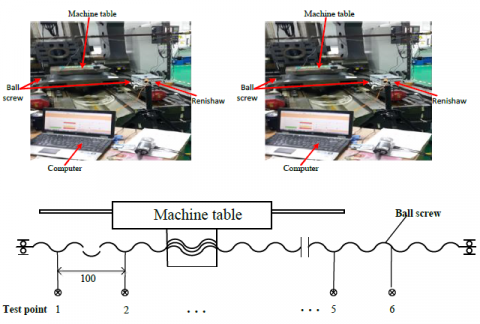Figure 4. Test bench of double-drive feed system

Figure 4 shows the test bench, which comprises the mechanical part, the temperature measurement part, and the feed system axial displacement measuring device and the control-display part.

After the temperature of the test point of the machine tool feed system reached the thermal equilibrium of 40℃, the results of this experiment were obtained by comparison with the same position in the two ball screws. The maximum value of temperature T1, T2, T3, T4 and the maximum deformation are shown in Table 1.

The speed of the ball screw is 500rpm. The temperature of the measuring points from ball screw 1 is larger than that of ball screw 2. The influence of thermal-mechanical coupling on the structural deformation is more important than simply considering the deformation caused by force or heat respectively. Under the same working conditions in this experiment, according to the simulation model of the feed system of machine tool, the simulation model of the ball screw 1 is established in this chapter. The results are shown in Table 2 and Table 3.

Table 1. Experimental results of feed system

 Test code T1 T2 T3 T4 s Parameter index Temp [℃] Temp [℃] Temp [℃] Temp [℃] D [um] screw 1 40.3 35.5 27.9 35.9 8.5

Table 2. Results of thermal-force coupling

 Sensor code Parameter index Thermal-force coupling Experimental value Calculated value Deviation value T1 Temp[℃] 39.4 41.5 5.33% T2 Temp[℃] 34.8 37.2 6.89% T3 Temp[℃] 26.9 28.7 6.69% T4 Temp[℃] 34.7 32.3 -6.92% T5 Temp[℃] 25.9 27.1 4.63% T6 Temp[℃] 26.8 25.5 -4.85% s Deformation [μm] 10.9 10.2 -6.42%

Table 3. Results of  without considering the coupling

 Sensor code Parameter index Without considering the coupling Experimental value Calculated value Deviation value T1 Temp[℃] 36.1 34.9 -3.33% T2 Temp[℃] 31.2 33.1 6.09% T3 Temp[℃] 25.3 26.8 5.92% T4 Temp[℃] 30.8 29.1 -5.52% T5 Temp[℃] 25.8 26.9 4.26% T6 Temp[℃] 26.2 24.9 -4.96% s Deformation [μm] 5.8 5.3 -8.62%

It can be seen from Table 2 and Table 3 that the temperature values, deformation values and relative errors between the experimental values and simulation model values are not great, which also further proves that the simulation modeling and analysis methods of thermal-mechanical coupling of the machine tool’s feed system in this paper are rational and feasible.

In order to explain the effect of heat on the dynamic performance of the feed system, a vibration experiment of the system was carried out to measure the amplitude and natural frequency of the system. The test system is shown in Figure 5.

## 5.png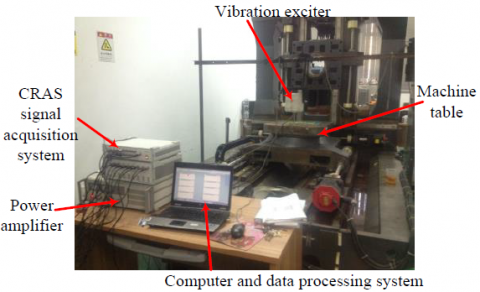Figure 5. Dynamic test system of double-drive feed system

4. Results and Discussion

4.1 Steady analysis of thermal-mechanical coupling vibration of feed system

Table 4 shows that the natural frequency of feed system is reduced with the heat impact of the screw. With the thermal deformation of the screw, the tensile force is reduced, but the stiffness of the screw and the frequency are decreased.

Table 4. Comparison of 1 to 6 Frequency

 Natural frequency 1 2 3 4 5 6 Initial state[Hz] 112 140 172 252 296 330 Thermal equilibrium[Hz] 104 128 166 250 286 328

The dynamic experimental amplitude of the feed system under the initial condition (a1, b1, c1) and thermal equilibrium (a2, b2, c2) are shown in Figure.6. Compared with the results of the finite element calculation and experiments, the errors are not great, so the accuracy of the analysis is reasonable. It can also be clearly seen that the amplitude of the thermal equilibrium is significantly higher than that of the initial state in the x, y, z directions for the feed system.

## 6.pngFigure 6. Amplitude comparison between static and thermal balance of double-drive feed system

4.2 Transient analysis of the thermal coupling vibration of the feed system

As shown in Figure 7, the transient analysis of the feed system coupled with the thermal-mechanical vibration includes the formulation of transient analysis time, the simulation of the transient process and the result of thermal mechanical coupling. Four coupling steps as shown in Table 5 have been selected for dynamic analysis of transient thermal coupling, and the results are shown in Figure 8.

## 7.png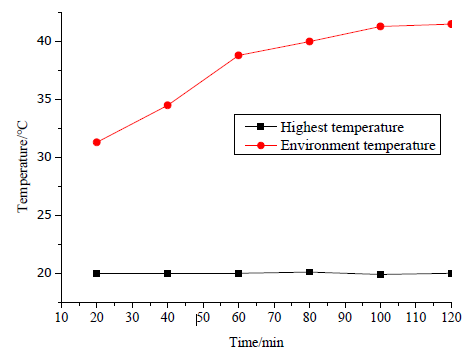Figure 7. Highest temperature of double-drive feed system rising process

Table 5. Thermal-mechanical coupling time steps

 Coupling time step 1 2 3 4 Time note /min 40 60 80 100

## 8.png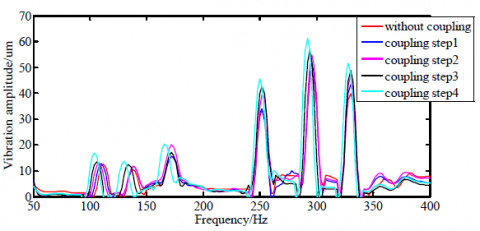Figure 8. Transient dynamic amplitude contrast

As shown in Figure. 8, the vibration amplitude increased with the increase in temperature. When the frequency is higher, this phenomenon is more obvious. The heat source is mainly caused by the ball screw rotation of the bearing before the thermal balance. The above analysis results show that before the double-drive feed system reaches a thermal steady state, as its thermal energy continually transforms into mechanical energy, the vibration amplitude increases, with the maximum rate of increase up to 14.06%. As a consequence, the effect of thermal-mechanical coupling must be considered in the process of optimizing the structure of the feed system.

5. Conclusions

Regarding the problem of a double-drive ball screw feed system, this paper studies the thermal-mechanical coupling of a double-drive system, and analyzes its dynamic characteristics and thermal characteristics. The simulation modeling and analysis method of thermal-mechanical coupling of the machine tool’s feed system in this paper are rational and feasible. From the results, the following conclusions can be drawn:

(1) Temperature increase and deformation between the ball screws 1 and 2 are different. The temperature of measuring points from ball screw 1 is greater than that of ball screw 2.

(2) The frequency of the double-drive feed system can be increased without considering the thermal mechanical coupling. The first order natural frequency increased from 104Hz to 112Hz and second order natural frequency increased from 128Hz to 140Hz.

(3) The thermal deformation can cause pre-tension deformation of the ball screwto a lesser extent, and the amplitude of the system is increased by 14.06%.

(4) The study of the influence of thermal-mechanical coupling on a double-drive feed system provides an important theoretical basis for multi-objective optimization design of the double-drive feed system of machine tools.

Acknowledgment

This project is supported by the natural science foundation of Jiangsu Province (BK20141400), and Key Special Project of Numerical Control Machine Tool (2013ZX04002-001 and 2015ZX04014021).

Nomenclature
 [Ct] specific heat capacity matrix {T} node temperature vector [Kt] heat conduction matrix {Q} heat flux vector Kijε the element in the domain Hijε the modification of the heat transfer matrix with the boundary conditions {T} node temperature vector Nε interpolation function Ωε solution domain S3ε third boundary conditions S2ε second boundary conditions q heat flux, W.m-2 Tα environment temperature qv heat flow in the solution domain k the thermal conductivity of materials C the specific heat, J.kg-1.K-1 ex strain in x direction ey strain in y direction ez strain in z direction

Greek symbols

 λx thermal conductivity, W.m-1. K-1 α thermal diffusivity, m2. s-1 E the elastic modulus μ Poisson ratio ν a thermal-mechanical coupling factor
References

 Kim D.I., Jung S.C., Lee J.E., Chang S.H. (2006). Parametric study on design of composite-foam-resin concrete sandwich structures for precision machine tool structures, Composite Structures, Vol. 75, No. 1, pp. 408-414. DOI: 10.1016/j.compstruct.2006.04.022

 Ramesh R., Mannan M.A., Poo A.N. (2000). Error compensation in machine tools — a review: part I: geometric, cutting-force induced and fixture-dependent errors, International Journal of Machine Tools and Manufacture, Vol. 40, No. 9, pp. 1235-1256. DOI: 10.1016/S0890-6955 (00)00009-2

 Liu S.H., Ye W.H. (2013). Dynamic analysis on feed system of gantry machine tool considering thermal–mechanical coupling, Journal of the Chinese Society of Mechanical Engineers, Vol. 34, No. 1, pp. 177-185.

 Li Y., Li Y.X., Yang M.S., Yuan Q., Cui F. (2015). Analysing the thermal mechanical coupling of 40Cr cold roll-beating forming process based on the Johnson–cook dynamic constitutive equation, International Journal of Heat and Technology, Vol. 33, No. 3, pp. 51-58. DOI: 10.18280/ijht.330307

 Giannetti N., Rocchetti A., Saito K. (2016). Thermodynamic optimization of three-thermal irreversible systems, International Journal of Heat and Technology, Vol. 34, Special Issue 1, pp. S83-S90. DOI: 10.18280/ijht.34S110

 Hesireh M.F., Yao W.S., Chiang C.R. (2007). Modeling and synchronous control of a single-axis stage driven by dual mechanically-coupled parallel ball screws, International Journal of Advance Manufacture Technology, Vol. 34, No. 9, pp. 933-943. DOI: 10.1007/s00170-007-1135-4

 Tian Y., Shirinzadeh B., Zhang D. (2009). A flexure-based five-bar mechanism for micro/nano manipulation, Sensors and Actuators A: Physical, Vol. 153, No. 1, pp. 96-104. DOI: 10.1016/j.sna.2009.04.022

 Yang J., Zhao D.H., Mei X.S., Zhao L., Ma C. (2015). Thermal error simulation and compensation in a jig-boring machine equipped with a dual-drive servo feed system, Pro IMechE: PartB Engineering Manufacture, Vol. 229, No. 51, pp. 43-63. DOI: 10.1177/0954405414555592

 Heisel U., Koscsak G., Stehle T. (2006). Thermography-based investigation into thermally induced positioning errors of feed drives by example of a ball screw, CIRP Annals: Manufacturing Technology, Vol. 55, No. 1, pp. 423-426. DOI: 10.1016/S0007-8506 (07)60450-8

 Liu S., Ye W., Lou P., et al. (2012). Bionic design for column of gantry machining centre to improve the static and dynamic performance, Shock and Vibration, Vol. 19, No. 4, pp. 493-504.

 Yen J., Chang H. (2004). Performance robustness and stiffness analysis on a machine tool servo design, International Journal of Machine Tools and Manufacture, Vol. 44, No. 5, pp. 523-531. DOI: 10.1016/j.ijmachtools.2003.10.024

 Kim M.S., Chung S.C. (2006). Integrated design methodology of ball-screw driven servo mechanisms with discrete controllers. Part I. modelling and performance analysis, Mechatronics, Vol. 16, No. 8, pp. 491-502. DOI: 10.1016/j.mechatronics.2006.01.008

 Zhu M., Mao K., Li B., Xiao W. (2014). An analytical method to select spindle speed variation parameters for chatter suppression in NC machining, Journal of Vibroengineering, Vol. 16, No. 1, pp. 447-463.

 Sarhan A.A.D. (2014). Investigate the spindle errors motions form thermal change foe high-precision CNC machining capability, International Journal of Advanced Manufacturing Technology, Vol. 70, No. 5, pp. 957-963. DOI: 10.1007/s00170-013-5339-5

 Karpat Y., Ozel T. (2008). Analytical and thermal modeling of high-speed machining with chamfered tools, Journal of Manufacturing Science and Engineering, Vol. 130, No. 1, pp. 119-129. DOI: 10.1115/1.2783282

 Stainier L., Ortiz M. (2010). Study and validation of a variational theory of thermo-mechanical coupling in finite visco-plasticity, International Journal of Solids and Structures, Vol. 47, No. 5, pp. 705-715. DOI: 10.1016/j.ijsolstr.2009.11.012